# Repeat patterns

The remaining patterns number 2616 are all wallpaper patterns and repeat in one of 17 ways. The most important characteristic is that of rotations. Hence we divide these patterns according to the maximal rotation present.

The first group are examples of patterns with no rotations. An example of two of these are: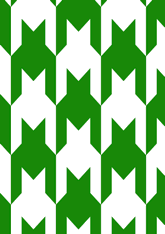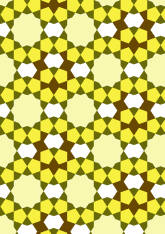The number in this group is 60, which is 2% of the total. For the next question, see page.
The second group are examples of patterns with rotations of order 2. An example of two of these are: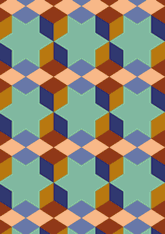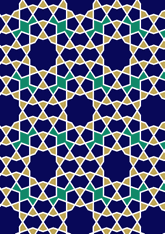Note that some pattern have rotations of order 2 and 4 - these are excluded here. The number in this group is 559, which is 21% of the total. For the next question, see page.
The third group are examples of patterns with rotations of order 3. An example of two of these are: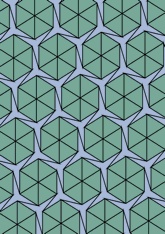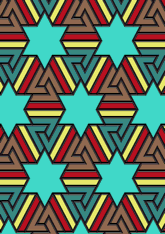Note that some pattern have rotations of order 3 and 6 - these are excluded here. The number in this group is 67, which is 2% of the total. For the next question, see page.
The fourth group are examples of patterns with rotations of order 4. An example of two of these are: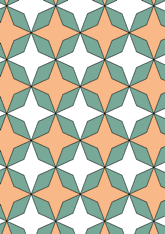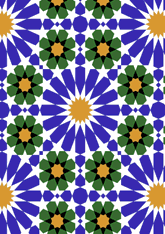The number in this group is 1310, which is 50% of the total. For the next question, see page.
The fifth group are examples of patterns with rotations of order 6. An example of two of these are: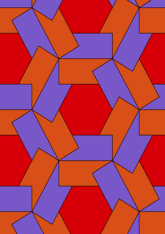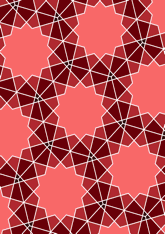The number in this group is 620, which is 23% of the total. For the next question, see page.

v53

Tiling Search was created by Brian Wichmann, and is maintained by MIT Libraries, through support from the Aga Khan Documentation Center.

Also supported by Performant Software Solutions LLC.